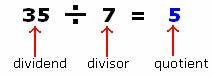Definition Of Quotient

When one number (dividend) is divided by another number (divisor), the result obtained is known as Quotient.

The quotient can be expressed as the number of times the divisor divides the dividend.

Examples of QuotientHere, when 35 ÷ 7, the quotient would be 5, while 35 would be called the dividend, and 7, the divisor.
The number 16, when divided by 3, has a quotient of 5 and the remainder as 1.

A. 7
B. 8
C. 6
D. 9